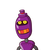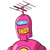# What is the formula to find the rate of discount when M.P and S.P after discount is given.

What is the formula to find the rate of discount when M.P and S.P after discount is given.

### 2 thoughts on “What is the formula to find the rate of discount when M.P and S.P after discount is given.<br />”

1.The discount is given in percentage. Hence, the M.P. is taken as Rs 100. Rate of discount = 5%. Amount of discount = (5/100)×100 = Rs 5.

2.Step-by-step explanation:

$$s.p = \frac{100 \: – \: d\%}{100} \times m.p$$

$$\frac{s.p}{m.p} \times 100 = 100 – d\%$$

$$d\% = 100 – ( \frac{s.p}{m.p} \: \times 100)$$

PLS MARK ME AS BRAINLIST